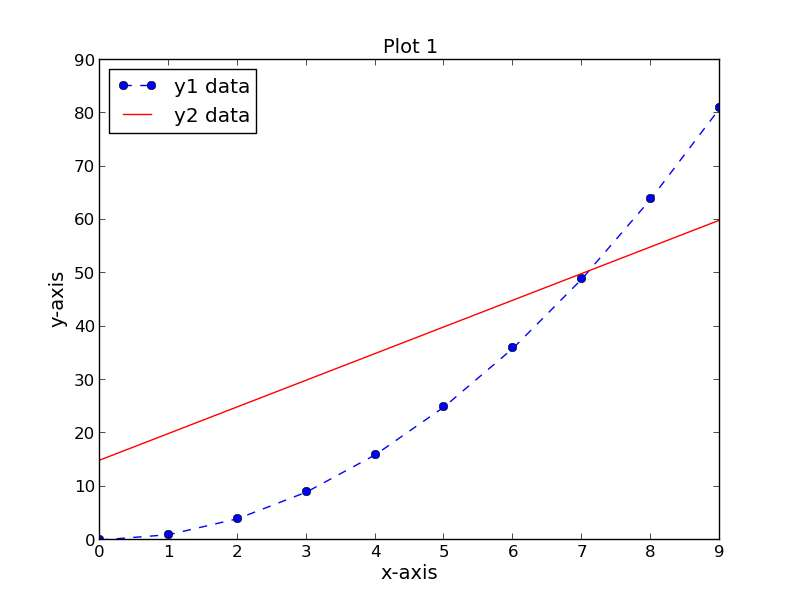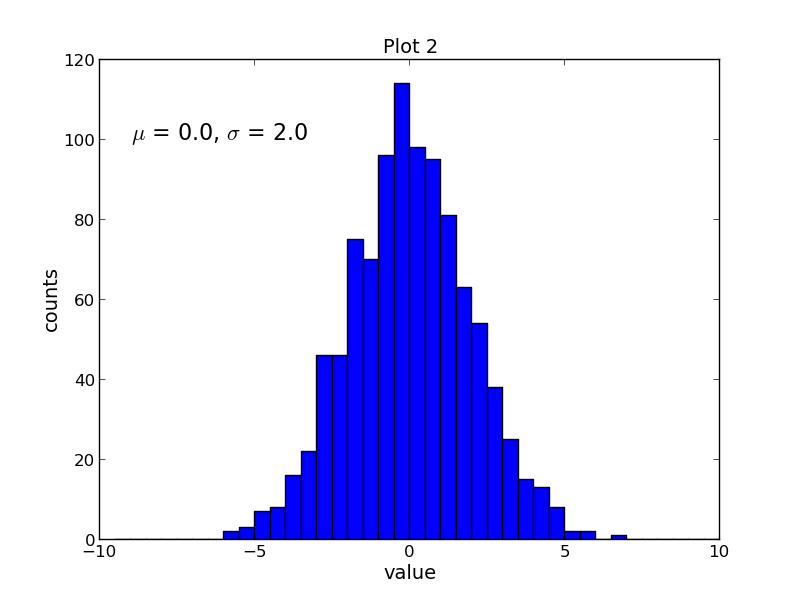# How to use matplotlib for scientific plotting on Linux

Last updated on August 24, 2020 by Dan Nanni

If you want an efficient, automatable solution for producing high-quality scientific plots in Linux, then consider using matplotlib. Matplotlib is a Python-based open-source scientific plotting package with a license based on the Python Software Foundation license. The extensive documentation and examples, integration with Python and the NumPy scientific computing package, and automation capability are just a few reasons why this package is a solid choice for scientific plotting in a Linux environment. This tutorial will provide several example plots created with matplotlib.

## Features

• Numerous plot types (bar, box, contour, histogram, scatter, line plots...)
• Python-based syntax
• Integration with the NumPy scientific computing package
• Source data can be Python lists, Python tuples, or NumPy arrays
• Customizable plot format (axes scales, tick positions, tick labels...)
• Customizable text (font, size, position...)
• TeX formatting (equations, symbols, Greek characters...)
• Compatible with IPython (allows interactive plotting from a Python shell)
• Automation - use Python loops to iteratively create plots
• Save plots to image files (png, pdf, ps, eps, and svg format)

The Python-based syntax of matplotlib serves as the foundation for many of its features and enables an efficient workflow. There are many scientific plotting packages that can produce quality plots, but do they allow you to do it directly from within your Python code? On top of that, do they allow you to create automated routines for iterative creation of plots that can be saved as image files? Matplotlib allows you to accomplish all of these tasks. You can now look forward to saving time that would have otherwise been spent manually creating multiple plots.

## Installation of matplotlib

Installation of Python and the NumPy package is a prerequisite for use of matplotlib. Instructions for installing NumPy can be found here.

To install matplotlib in Debian or Ubuntu, run the following command:

```\$ sudo apt-get install python-matplotlib
```

To install matplotlib in Fedora or CentOS/RHEL, run the following command:

```\$ sudo yum install python-matplotlib
```

## Matplotlib Examples

This tutorial will provide several plotting examples that demonstrate how to use matplotlib:

• Scatter and line plot
• Histogram plot
• Pie chart

In these examples we will use Python scripts to execute matplotlib commands. Note that the numpy and matplotlib modules must be imported from within the scripts via the `import` command. `np` is specified as a reference to the `numpy` module and `plt` is specified as a reference to the `matplotlib.pyplot` namespace:

```import numpy as np
import matplotlib.pyplot as plt
```

## Example 1: Scatter and Line Plot

The first script, `script1.py` completes the following tasks:

• Creates three data sets (`xData`, `yData1`, and `yData2`)
• Creates a new figure (assigned number 1) with a width and height of 8 inches and 6 inches, respectively
• Sets the plot title, x-axis label, and y-axis label (all with font size of 14)
• Plots the first data set, `yData1`, as a function of the `xData` dataset as a dotted blue line with circular markers and a label of "y1 data"
• Plots the second data set, `yData2`, as a function of the `xData` dataset as a solid red line with no markers and a label of "y2 data".
• Positions the legend in the upper left-hand corner of the plot
• Saves the figure as a PNG file

Contents of `script1.py`:

```import numpy as np
import matplotlib.pyplot as plt

xData = np.arange(0, 10, 1)
yData1 = xData.__pow__(2.0)
yData2 = np.arange(15, 61, 5)
plt.figure(num=1, figsize=(8, 6))
plt.title('Plot 1', size=14)
plt.xlabel('x-axis', size=14)
plt.ylabel('y-axis', size=14)
plt.plot(xData, yData1, color='b', linestyle='--', marker='o', label='y1 data')
plt.plot(xData, yData2, color='r', linestyle='-', label='y2 data')
plt.legend(loc='upper left')
plt.savefig('images/plot1.png', format='png')
```

The resulting plot is shown below:## Example 2: Histogram Plot

The second script, `script2.py` completes the following tasks:

• Creates a data set containing 1000 random samples from a Normal distribution
• Creates a new figure (assigned number 1) with a width and height of 8 inches and 6 inches, respectively
• Sets the plot title, x-axis label, and y-axis label (all with font size of 14)
• Plots the data set, `samples`, as a histogram with 40 bins and an upper and lower bound of -10 and 10, respectively
• Adds text to the plot and uses TeX formatting to display the Greek letters `mu` and `sigma` (font size of 16)
• Saves the figure as a PNG file

Contents of `script2.py`:

```import numpy as np
import matplotlib.pyplot as plt

mu = 0.0
sigma = 2.0
samples = np.random.normal(loc=mu, scale=sigma, size=1000)
plt.figure(num=1, figsize=(8, 6))
plt.title('Plot 2', size=14)
plt.xlabel('value', size=14)
plt.ylabel('counts', size=14)
plt.hist(samples, bins=40, range=(-10, 10))
plt.text(-9, 100, r'\$mu\$ = 0.0, \$sigma\$ = 2.0', size=16)
plt.savefig('images/plot2.png', format='png')
```

The resulting plot is shown below:## Example 3: Pie Chart

The third script, `script3.py` completes the following tasks:

• Creates data set containing five integers
• Creates a new figure (assigned number 1) with a width and height of 6 inches and 6 inches, respectively
• Adds an axes to the figure with an aspect ratio of 1
• Sets the plot title (font size of 14)
• Plots the data set, `data`, as a pie chart with labels included
• Saves the figure as a PNG file

Contents of `script3.py`:

```import numpy as np
import matplotlib.pyplot as plt

data = [33, 25, 20, 12, 10]
plt.figure(num=1, figsize=(6, 6))
plt.axes(aspect=1)
plt.title('Plot 3', size=14)
plt.pie(data, labels=('Group 1', 'Group 2', 'Group 3', 'Group 4', 'Group 5'))
plt.savefig('images/plot3.png', format='png')
```

The resulting plot is shown below:## Summary

This tutorial provides several examples of plots that can be created with the matplotlib scientific plotting package. Matplotlib is a great solution for scientific plotting in a Linux environment given its natural integration with Python and NumPy, its ability to be automated, and its production of a wide variety of customizable high quality plots. Documentation and examples for the matplotlib package can be found at the official site.

## Support Xmodulo

This website is made possible by minimal ads and your gracious donation via PayPal or credit card